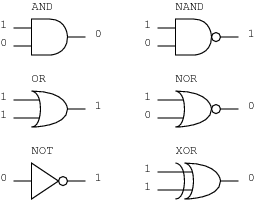Tutorial Intro to CPU design [Part 2: Logic and boolean algebra] filter_list Author Message
Intro to CPU design [Part 2: Logic and boolean algebra] #1

Alright, so we know now how to deal with binary numbers and hexadecimal numbers. We know that 1+1=10. This tutorial is where I get to confuse the crap out of you.

1+1=1
Boolean algebra works differently than binary number systems. Boolean algebra works bit by bit, and uses logic operations instead of our normal add, subtract, multiply, etc.

Let me simply name the logic operations we will cover in this tutorial. There are MANY more operations, but we will stick to the basics.
• OR
• AND
• NOT
• NOR
• NAND
• XOR
• XNOR

So, let's begin now:

OR
Or is a logic operation that takes two arguments (A and B) and returns logic HIGH (binary 1) if either A OR B are at logic HIGH.
For example, here is a table:
X= A OR B
Code:
```A|B|X 0|0|0 0|1|1 1|0|1 1|1|1```
Seems pretty simple. Cool.

AND
AND is a logic operation much like OR, but will only return HIGH if both A AND B are at logic high. Here is the table:
X=A AND B
Code:
```A|B|X 0|0|0 0|1|0 1|0|0 1|1|1```
Oh look, another easy one.

NOT
The NOT operation is the easiest one so far (yes, there is one easier than the NOT gate). All it does, is take 1 argument (A) and return the opposite of it.
X=NOT A
Code:
```A|X 0|1 1|0```

NOR
NOR is NOT OR, literally it is the same as the following operation:
X = A OR B
Y = NOT X
therefore
Y also = A NOR B
Here is the table
X = A OR B
Y = A NOR B
Code:
```A|B|X|Y 0|0|0|1 0|1|1|0 1|0|1|0 1|1|1|0```
It really isn't too difficult to understand, just take the output from an OR operation, and take the opposite.

NAND
NAND is the same concept of NOR, except it is just NOT AND.
Table:
X = A AND B
Y = A NAND B
Code:
```A|B|X|Y 0|0|0|1 0|1|0|1 1|0|0|1 1|1|1|0```
Once again, just an AND, and then the opposite (NOT) of that

XOR
XOR, or exclusive-or, is one of the tricky ones. It is simply: A OR B, but NOT A AND B. Basically, it will be logic HIGH if A and B are different, and logic LOW if they are the same.
For those of you who are catching on quickly, it actually means
(A AND (NOT B)) OR (B AND (NOT A))
Here is the table:
X=A XOR B
Code:
```A|B|X 0|0|0 0|1|1 1|0|1 1|1|0```
It might be a little complex, but I know if you read over it a couple times you will understand.

XNOR
XNOR, or NOT exclusive or, is just a not placed onto an exclusive or, causing it to read logic HIGH when the values are the same, and logic LOW when different:
X=A XNOR B
Code:
```A|B|X 0|0|1 0|1|0 1|0|0 1|1|1```

There you have it, all of the gates we will be using in our CPU. Now, we have to cover notation:
Back to our list.
OR
AND
NOT
NOR
NAND
XOR
XNOR

Now, some of these don't have notation symbols, so let's revise our list, and i will write down the symbols:
OR = +
AND = * (or two variables next to each other, like AB is the same as A*B)
NOT = ' (or a line drawn above the variable name)
XOR = a plus with a circle drawn around it. It can also be written AB'+A'B

Here is an image I found that shows the drawings for each of these gates. The only one missing is the XNOR, which is just the XOR with the little circle after it like the NOR gate has.Alright, next up is probably going to be a tutorial on writing boolean logic equations. We will use the graphical method, so there will be a lot of images, it will be mostly handwritten.

Thoughts?

Users browsing this thread: 1 Guest(s)